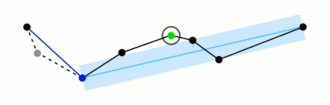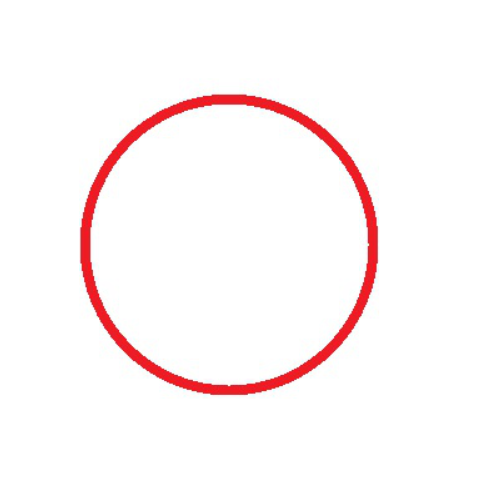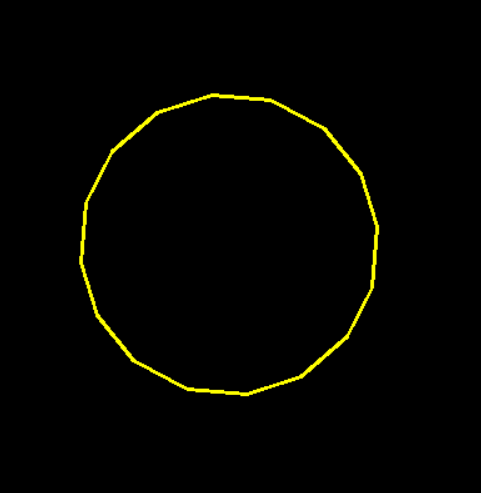# Detailed explanation of OpenCV approxpolydp() function

``````CV_EXPORTS_W void approxPolyDP( InputArray curve,OutputArray approxCurve,double epsilon, bool closed );

@param curve Input vector of a 2D point stored in std::vector or Mat
@param approxCurve Result of the approximation. The type should match the type of the input curve.
@param epsilon Parameter specifying the approximation accuracy. This is the maximum distance
between the original curve and its approximation.
@param closed If true, the approximated curve is closed (its first and last vertices are
connected). Otherwise, it is not closed.

The function cv::approxPolyDP approximates a curve or a polygon with another curve/polygon with less
vertices so that the distance between them is less or equal to the specified precision. It uses the
Douglas-Peucker algorithm <http://en.wikipedia.org/wiki/Ramer-Douglas-Peucker_algorithm>``````

The main function is to crease a continuous smooth curveThere are four parameters

Inputarray curve: input curve. The data type can be vector & lt; point & gt;.

Outputarray approxcurve: output polyline. The data type can be vector & lt; point & gt;.

Double epsilon: the threshold value for judging the distance between the point and the corresponding line segment. (if the distance is greater than this threshold, it will be discarded. If the distance is less than this threshold, it will be retained. The smaller the epsilon is, the closer the shape of the broken line is to the curve.)

Bool closed: Flag of whether the curve is closed or not.

Program example:

``````#include<opencv2/opencv.hpp>
#include<iostream>

using namespace std;
using namespace cv;

void main()
{
Mat srcImg = imread("01.jpg");
imshow("src", srcImg);
Mat dstImg(srcImg.size(), CV_8UC3, Scalar::all(0));

cvtColor(srcImg, srcImg, CV_BGR2GRAY);
threshold(srcImg, srcImg, 200, 255, CV_THRESH_BINARY_INV);
vector<vector<Point>> contours;
vector<Vec4i> hierarcy;
findContours(srcImg, contours, hierarcy, 0, CV_CHAIN_APPROX_NONE);
vector<vector<Point>> contours_poly(contours.size());
for (int i = 0; i<contours.size(); i++)
{
approxPolyDP(Mat(contours[i]), contours_poly[i], 15, true);

drawContours(dstImg, contours_poly, i, Scalar(0, 255, 255), 2, 8);
}
imshow("approx", dstImg);
waitKey(0);
}``````

Image “01. JPG”:Epsilon was 15Epsilon was 5